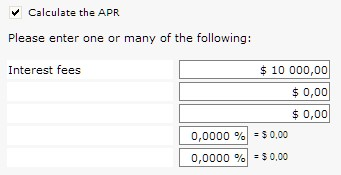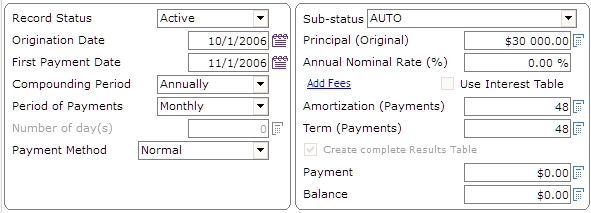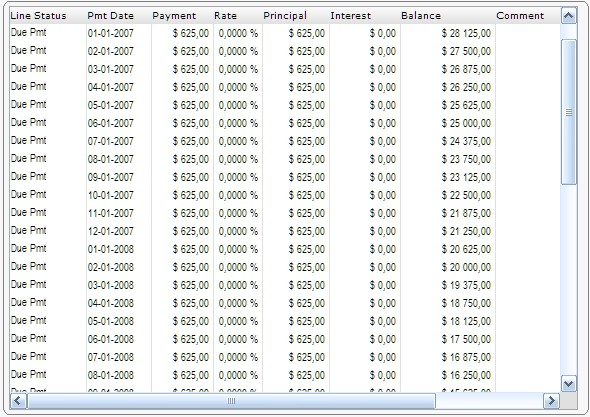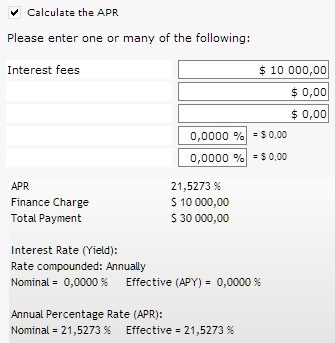Create a new Record with “File” and “New Record.”“Add-on interest” is a calculation that Margill Loan Manager (MLM) handles easily to compute the true interest rate (APR).

For example, a car dealer advertises a rate of \$12.50 per \$100 per year on a \$20,000 loan to be repaid in monthly payments over 4 years. (*While this example is in dollars, Margill can calculate interest in any currency.)

How much interest is payable? What is the interest rate (or APR) on this loan?

## APR window

This will give us a total add-on of \$10,000 in interest: \$20,000/100 X \$12.50 X 4. This \$10,000 is added in the APR window.## Data window

The total loan is thus \$30,000 to be repaid in 48 equal payments at “0.00%” interest. The compounding period is not important since for reimbursement, the interest rate is 0.00%.Once the data is entered, press “Compute.” The payment will be calculated by MLM (\$625 per month) and the detailed payment schedule produced:To obtain the APR, click on “APR.” The rate calculated is 21.5273% (thus the APR).